##### Macroeconomics For Dummies - UK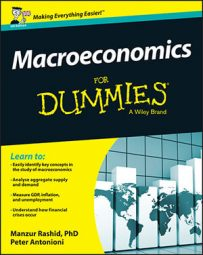Many people prefer to avoid equations, but the ones described below are vital to understanding macroeconomics. So, take a look! They’ve proved themselves immensely useful over the years.

## Production function

Y = f(K, L)

The production function says that a nation’s output depends upon two things:

• The available factors of production (K, L).

• How good the technology (f) is at turning inputs (K, L) into output, Y.

This simple equation means that if an economy is to grow, it either needs to increase the quantity/quality of its factors of production or improve upon its technology. One way of improving technology is by incentivising firms to engage in research and development (R&D), thus creating new ways of making things that people want to buy.

## Fisher equation

i = r + π

The Fisher equation links the nominal interest rate (i), the real interest rate (r) and the rate of inflation (π). So, for example, if your bank is offering you a return of 10 per cent (yeah, right!) and inflation is running at 6 per cent, your real return is 4 per cent.

Economists think the real interest rate is what counts, and because nominal things such as money can’t affect real things in the long run, monetary policy can’t affect the real interest rate in the long run. In fact, increasing inflation by 1 per cent increases the nominal interest rate by 1 per cent as well ‒ and this result is the Fisher effect.

## Breaking down output

Y = C + I + G + NX

This equation breaks output (Y) into its constituent components:

• Consumption (C): The largest component, comprising household expenditure on goods and services.

• Investment (I): Firms’ purchase of capital goods (such as machines and factories).

• Government expenditure (G): Government spending on goods and services.

• Net exports (NX): Exports minus imports, representing demand for goods and services from abroad.

## Closed economies

S = I

This equation says that in a closed economy (one that doesn’t trade with the rest of the world) savings (S) must equal investment (I).

This relationship is important, because often commentators talk about the importance of boosting consumption for the health of the economy. But this logic makes little sense in the long run: If you want the economy to grow in the long run, you want less consumption and more saving. This is because more saving leads to more investment, which means more capital, which means more future output.

## Open economies

S – I = NX

This equation is the corresponding relationship between investment (I) and saving (S) in an open economy (one that trades with the rest of the world).

The equation has an important interpretation. If the level of savings (S) in an economy is greater than the demand for investment (I), this excess savings (SI) flows abroad (which is why SI is called net capital outflow). The equation tells you that this net capital outflow will exactly equal net exports (NX). Therefore, if the UK is a net exporter to the rest of the world (NX > 0), the UK must have lent exactly the right amount of money (SI) for foreigners to make this purchase. How convenient!

## Quantity equation

MV = PY

The quantity equation says that the amount of money in an economy (M) multiplied by how fast money circulates (V) is always equal to the price level (P) multiplied by real output (Y).

Why? Well, the left-hand side measures the total value of purchases in an economy (its nominal GDP), which is exactly what the right-hand side measures too!

## Growth rate of the money supply

gm = π + gy

This equation follows from the quantity one in the preceding section. It says that the growth rate of the money supply (gm) is equal to the rate of inflation (π) plus the growth rate of real GDP (gy); gy is unaffected by monetary policy in the long run, so increasing the growth of the money supply leads to a one-for-one increase in inflation. This is what Friedman meant when he said, ‘Inflation is always and everywhere a monetary phenomenon’.

## Equation of motion for the capital stock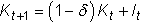This equation says that the capital stock tomorrow (Kt+1) will be today’s capital stock (Kt) less depreciation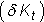plus investment today (It): that is, new capital stock.

It makes clear that the amount of investment today has to be more than the depreciation of the capital stock in order for the capital stock to increase.

## Government’s intertemporal budget constraint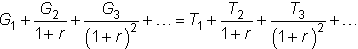Despite hardly tripping lightly off the tongue, the government’s intertemporal (across time) budget constraint says that the net present value of present and future government spending must always equal the net present value of present and future taxes.

In other words, even the government has to balance the books eventually.

## Phillips curve relationship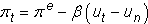The Phillips curve relationship says that in the short run, a negative relationship exists between inflation today (πt) and unemployment today (ut). Also, when actual inflation today (πt) equals expected inflation (πe), unemployment is equal to its natural rate (un).

So in the short run, reducing unemployment below its natural rate is possible, so long as you can surprise people by creating higher inflation than they expect.